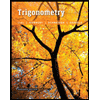# figure is a golden rectangle. It is constructed from square ACDB by holding line segment OB fixed at point o and then letting point B drop down untl oB te ratio of the length to the width in the golden rectangle is called the golden ratio. Find the lengths below to arive at the golden ratio. (Let a - 2.) is called the golden ratio. Find the lengths below to arrive at the golden ratio. (Let a 2.) 2a (a) Find the length of OB. (b) Find the length of OE. OE = (c) Find the length of CE. CE = (d) Find the ratio CE EF CE EF Need Help? Read It Talk to a Tutor

Question

thank you soo much

Expert Solution

### Want to see the full answer?

Check out a sample Q&A hereStudents who’ve seen this question also like:Trigonometry (11th Edition)
11th Edition
ISBN: 9780134217437
Author: Margaret L. Lial, John Hornsby, David I. Schneider, Callie Daniels
Publisher: PEARSON